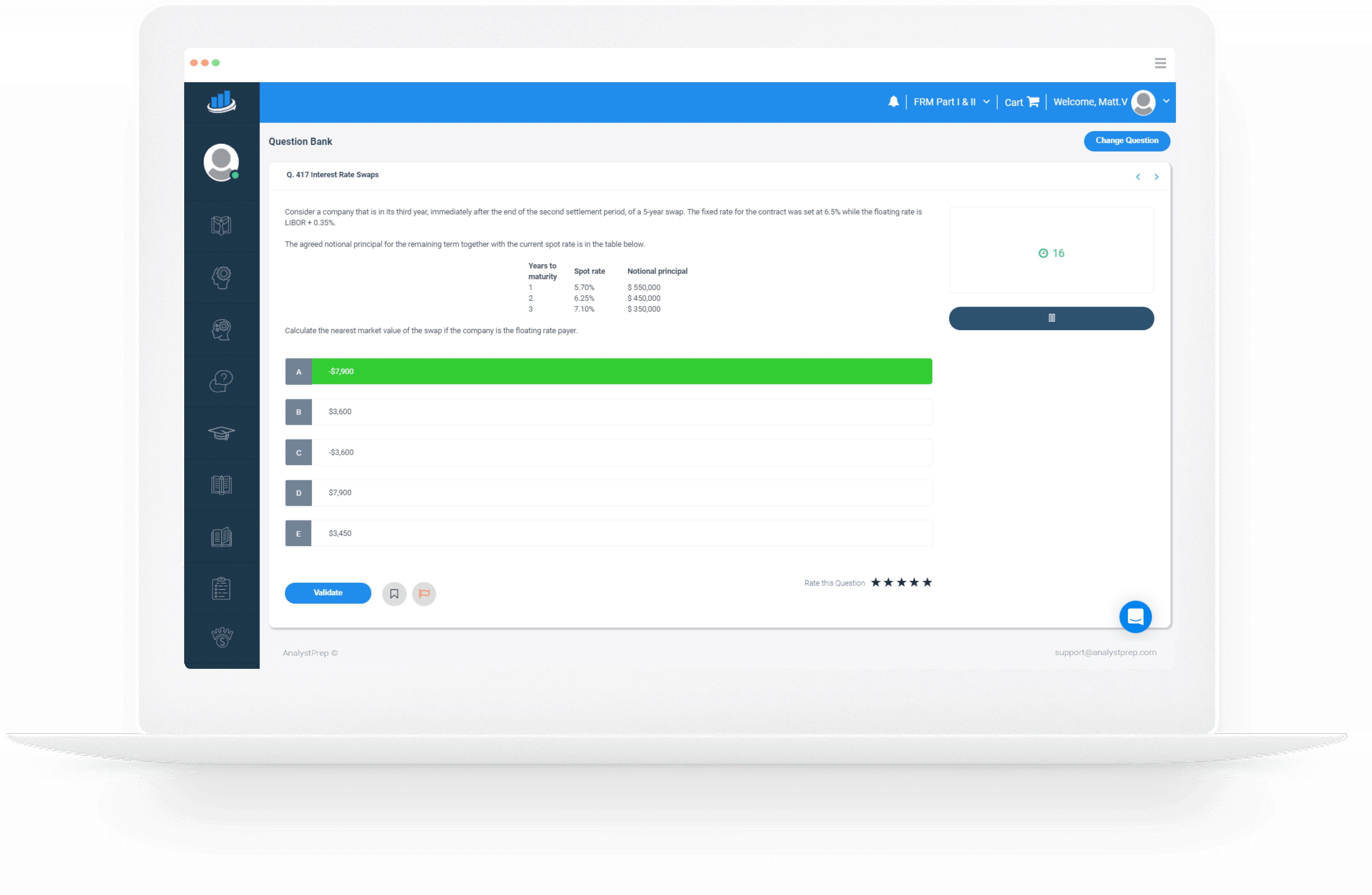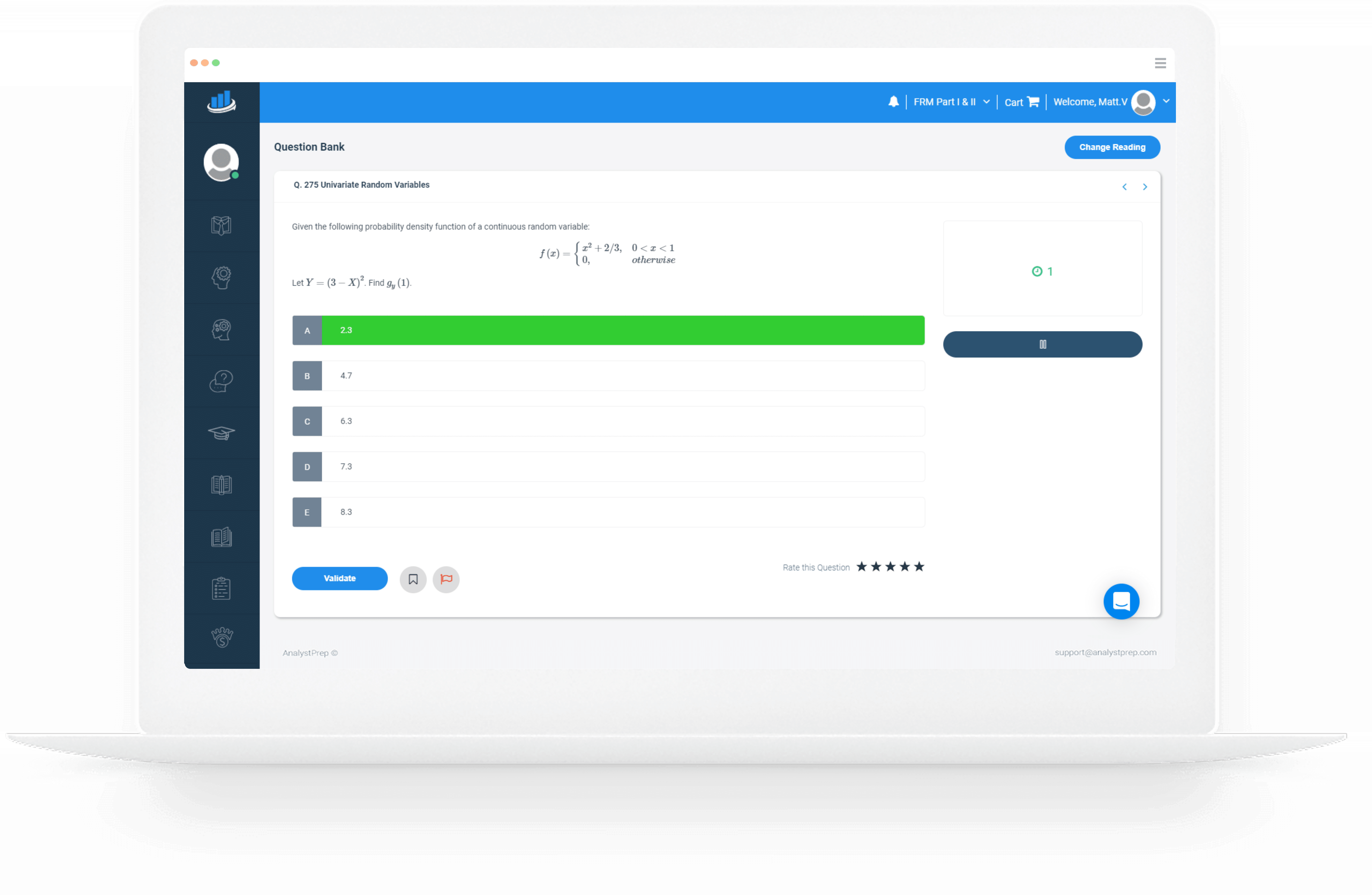Study Materials for 2023 CFA®, FRM®, Actuarial, GMAT® and EA® Exams

### SOA Exam FM Practice Questions# Financial Mathematics Question Bank

At AnalystPrep, our question bank is designed to further expand your knowledge of the application of financial mathematics aspects in determining the present and accumulated values for several streams of cash flows. Our financial mathematics practice questions (multiple-choice questions from A to E – just like the actual exam) reflect the difficulty and style of the Exam FM from the Society of Actuaries. We also do post regular updates to incorporate the latest review from our team of academics and actuaries.### How is the FM Question Bank Subdivided?

AnalystPrep’s question bank for Exam FM has been specifically crafted around the syllabus given by the Society of Actuaries. As such, it is subdivided into eight main topics:

• Time value of money (10-15%)
• Annuities/cash flows with non-contingent payments (15-20%)
• Loans (10-20%)
• Bonds (10-20%)
• General cash flows and portfolios (15-20%)
• Immunization (10-15%)
• Interest rate swaps (0-10%)
• Determinants of interest rates (0-10%)

However, a question and answer bank doesn’t mean you will revise and practice alone. Our team of expert instructors is always on hand to supplement the solutions provided with additional tips and tricks.

4.5  Million
50  Thousand
# 1  Rated

# Free Financial Mathematics Practice Problems with Answers

## Time Value of Money

An investment strategy achieves a nominal rate of 10%. The nominal rate is compounded once in year 1, twice in year 2, 3 times in year 3, etc. Calculate the amount that must be invested today in order to accumulate 5,000 after 4 years.

A) 2,450.23

B) 2,584.36

C) 2,584.25

D) 2,054.98

E) 3,385.18

Let the amount that must be invested today be $$X$$.

Then,
$$X \left( 1+ \frac {1}{10} \right) \left(1+\frac {1}{2 \ast 10} \right)^2 \left(1+ \frac {1}{3 \ast 10} \right)^3 \left(1+ \frac {1}{4 \ast 10} \right)^4=5000$$.

Solve for $$X$$:
$$X=3385.18.$$

Dave receives payment every year. The first payment is $10,000 now. In the subsequent years, the payment decreases by 6%. He will receive 10 payments in total. Assume an annual e?ective interest rate of 6%. What is the present value of the payments? A) 61,766.21 B) 79,380.29 C) 129,380.49 D) 249,380.29 E) 259,380.29 The correct answer is: A) This is a decreasing annuity-due in a geometric progression: $$10000\left( 1.06 \right) \frac { 1-{ \left( \frac { 0.94 }{ 1.06 } \right) }^{ 10 } }{ 0.06+0.06 } =61,766.21.$$ ## Question 204 ## Loans A loan of$480,000 is structured such that it is repayable over 25 years, by monthly payments in arrears of amount $2780. Calculate the flat rate of interest paid on the loan. A) 2.89% B) 2.56% C) 2.95% D) 2.87% E) 2.55% The correct answer is: C) The total amount of money paid is $$12 \times 2780 \times 25=834,000$$ $$\Rightarrow interest \quad paid =834,000-480,000=354,000$$ The flat rate of interest is given by: $$\frac {354,000}{480,000 \times 25}=0.0295=2.95\%$$ ## Question 280 ## Bonds A 10 year bond with a par value of$1,000 will mature in 10 years for $1,080. It sells at$1193.07 with yield 6% convertible semiannually. Find the coupon rate if it is convertible semiannually.

A) 4%

B) 5%

C) 6%

Ð) 8%

E) 10%

Using the premium/discount formula we have:
\begin{align*} P=C+(Fr-Ci){ a }_{ \overline { n| } @i } =1080+{ { \left( 1000\ast r -1080\ast 0.03 \right) }{ a }_{ \overline { 20| } @30\% }=1193.07. }\\ \end{align*}
Solve for $$r=0.04$$. Thus the coupon rate is 8% convertible semiannually.

The price of a bond now is 116.73 and the current yield is 5%. The modified duration of the bond is 8.14. The yield changes to 6.30%. Calculate the new price of the bond. A) 105.698 B) 135.628 C) 104.378 D) 114.237 E) 125.23 The correct answer is: C) We know that: $$P(i+\epsilon_i )=P(i)\left[1-v(i)\epsilon_i \right]$$ now, $$P(0.05)=116.73,v(i)=8.14$$ and $$\epsilon_i=6.30-5=1.30\%=0.0130$$ \begin{align*} \Rightarrow P(0.0630)&=116.73\left[1-8.14 \times 0.0130 \right] \\ & =116.73 \times 0.89418=104.378 \\ \end{align*} ## Question 362 ## Immunization At 1 July 2007, a company has a liability of &dollar;20,000 which must be paid on 1 Jan 2011 and a liability of &dollar;18,000 to be paid on 1 July 2013. Currently, the company holds assets whose present value is equal to that of the liabilities. The company wishes to immunize its position by investing in two zero coupon bonds $$X$$ and $$Y$$, whose terms are 4 and 7 years respectively at an effective rate of interest of 10%. Calculate $$X+Y$$. A) 24,562 B) 25,369 C) 24,488 D) 23,564 E) 28,754 The correct answer is: C) According to Redington immunization, the present value of the liabilities must be equal to that of the assets, $$\Rightarrow X+Y=20000v^{3.5}+18000v^6=24488$$ $$X+Y=24488 … (i)$$ Again, the Macaulay duration must be equal, $$\Rightarrow 4X+7Y=3.5×20000v^{3.5}+6 \times 18000v^6=111108$$ $$X+7Y=111108 … (ii)$$ Solving (i) and (ii) simultaneously, $$X=20103 \quad and \quad Y=4385$$ $$\Rightarrow X+Y=20103+4385=24488$$ ## Question 415 ## Interest Rate Swaps TCG, a listed company, issued debt finance to finance its expansion in the form of a floating rate bond. The face value of the bond is 650 m redeemable in five years with interest linked to LIBOR.

TCG wishes to protect itself against adverse movements in interest rates by taking an interest rate swap with the current spot rates as per the table below.

 Term (years) Spot rate 1 5.25% 2 6.10% 3 6.95% 4 7.25%

What fixed interest rate should TCG agree to?

A) 6.25%

B) 7.16%

C) 6.40%

D) 7.25%

E) 6.60%

 Term(years) Spot rate $$\bf {P_{t_i }}$$ 1 5.25% $$(1+0.0525)^{-1} = 0.95012$$ 2 6.10% $$(1+0.0610)^{-2} = 0.88832$$ 3 6.95% $$(1+0.0695)^{-3} = 0.81744$$ 4 7.25% $$(1+0.0725)^{-4} = 0.75581$$

$$\frac { 1-{ P }_{ { t }_{ 4 } } }{ \sum _{ i=1 }^{ 4 }{ { P }_{ { t }_{ i } } } } =\frac { 1-0.75581 }{ 3.41169 } =0.0716\quad i.e.\quad 7.16\%$$

## Determinants of Interest Rates

NewBank is a new bank that will only a 3-year bullet loan with all the principal and interest paid at the end of the term. The bank wants to receive an effective per annum interest rate of 6.15% before addition of any risk premiums.

If 1% of the borrowers are expected to default with the bank able to only recover 25% from these borrowers, estimate the default risk premium the bank needs to include in its pricing.

A) 0.002

B) 0.0065

C) 0.009

D) 0.0062

E) 0.0027

$${ 1.0615 }^{ 3 }=1.19608$$
Due to default, the expected contractual repayment $$x$$ is defined by the equation
$$x\times 0.99+0.01\times 0.25\times x=0.9925x$$
$$0.9925x=1.19608\Rightarrow x=1.2051$$
$${ 1.2051 }^{ { 1 }/{ 3 } }-1=0.06417\quad i.e.\quad6.417\%$$
$$6.417\%-6.15\%=0.267\%$$